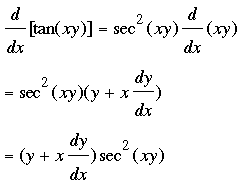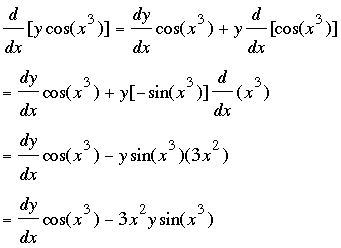SEARCH HOMEMath Central Quandaries & QueriesQuestion from mamiriri, a student: differentiate y sin[x^2]=x sin[y^2]Mamiriri,

Let me try a similar problem, tan(xy) = y cos(x3).

First let's look at the left side. You know the derivative of the tangent but you need also to apply the chain rule.Next look at the right side. It is the product of y and a cosine function. You know the product rule and the derivative of the cosine function but you need to apply the chain rule when you differentiate cos(x3).Set these two expressions equal and solve for dy/dx.